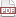### Another two-line Logit analysis

07 October 13. [link]PDF version

Today's post will not be especially intense: I point to a researcher doing some interesting things, complain about nomenclature, and give you a two-line example of a binomial model + logistic link using Apophenia.

Paul Gribble's lab studies the computational aspects of motor control. For example, his lab has a few papers on learning motor control by watching others. And here's an old pop writeup of this paper (PDF) on learning movement by observing. It's not my field, so I just read the abstracts and admire the march of science.

One of Paul's little side projects is a routine to estimate a Psychometric function, aka a binomial model + logit link function, aka a Logit.

So first, allow me a digression about nomenclature. A rose by any other name may smell as sweet, but it is more difficult to locate in the literature.

• psychometric function is a field-specific name, which leads to Balkanization, as every field that uses a Logit could develop a different name for the same thing.

• Binomial model + logistic link is a description of a specific form of the model. Using Paul's notation: $$y = \beta_0 + (\beta_1 * x)$$ $$p(x) = Pr(response|x) = 1 / (1 + exp(-y))$$ where $y$ is the observed outcome in the data, $x$ is the observed input, and the $\beta$s are parameters to be estimated. This is a reminder that we could change that second function to any of a number of other probability functions, another favorite being a simple Normal CDF (the Probit). Calling it a binomial model is a reminder that there are two options for the response. So this naming scheme generalizes somewhat, but it is also a certain way of thinking about the problem. For my tastes, it doesn't generalize far enough, being that it still presumes a fundamentally linear process, but I've already written that ten-part series of blog posts.

• Logit refers to a specific form, and doesn't really describe the model, except for the cutesy custom that models ending in -it (Logit, Probit, Tobit, Normit, …) are about categorical data. But Logit is just a vocabulary term, like magma or Markov chain, which you have to memorize. I think the name Logit came before the GLM-style binomial+logistic link form (where GLM=Generalized linear model).

I asked a librarian of Congress. She was furloughed and had nothing else to do. The task of a librarian is to store information so that it is easy to retrieve, meaning that your typical librarian is an expert in taxonomy and ontology. Of these options, she recommended the functional naming scheme, under the rationale that somebody who has no idea could conceivably find it. She suggested that the other options are less discoverable.

Naming something after a certain framework can be risky; we sometimes wind up with terms that made sense to the first author but are now a little confusing: regression, or Gamma distribution (which is based on, but does not behave like, the Gamma function). But digressions from the original meaning happen in every field (sneakers, cab, office, even computer).

Anyway, having identified the psychometric function Paul estimates as a Logit, it's pretty easy to estimate. Here's a shell script to cut and paste onto your command line, which will download his version and sample data and run it through Apophenia's Logit estimator.


mkdir logit_eg
cd logit_eg
git clone https://github.com/paulgribble/psychometric.git

cat >> makefile << '——'
CFLAGS =-g -Wall -O3  pkg-config --cflags apophenia
LDLIBS=pkg-config --libs apophenia
CC=c99
171-a_logit:
——

cat >> 171-a_logit.c << '——'
#include <apop.h>

int main(){
apop_text_to_db("psychometric/exdata", "d", .delimiters=" ", .has_col_names='n', .field_names=(char*[]){"measure", "outcome"});
apop_model_show(apop_estimate(apop_query_to_data("select outcome, measure from d"), apop_logit));
}
——

make && ./171-a_logit


The results are the same, though the methods of calculation are a little different. The Logit objective function is globally concave, which makes it a good candidate for gradient descent-type MLE search algorithms. Also, the Gribble psychometric edition adds some additional diagnostics and has some nice plots. Somebody could easily add some of those to Apohenia's Logit estimation routine….